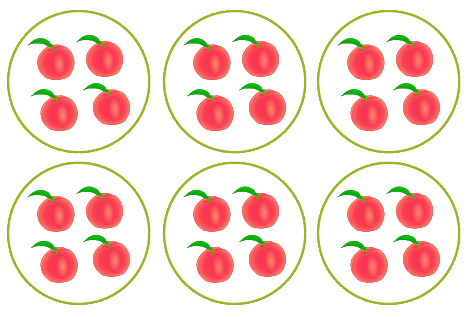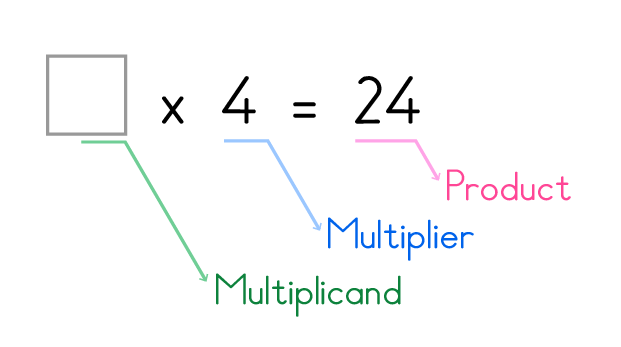# Write multiplication expression for equal groups - Understand Multiplication Concept

## 'Write multiplication expression for equal groups' Test and Printable Worksheets

The idea of kids to write multiplication expression for equal groups enables them to readily and easily understand multiplication concept.

This is because in our 'Write multiplication expression for equal groups' Test and Printable Worksheets we have exceptionally presented the exercises with pictures, and of course games bringing to kid's excitements.

Moreover, the exercises are very simple, though challenging.

## Click the sections below to find more explanation on this topic

...

### Know parts of multiplication expression - equal groups method

It is very easy to write multiplication expression for equal groups. The only secret here is to call to mind the parts of a multiplication expression.
It consist of three parts, namely multiplicand, multiplier and the product.

For example 6 x 3 = 18, where our first factor (6) is the multiplicand, second factor (3) the multiplier and our result (18), the product.

Whenever you find only two parts instead of three in a multiplication sentence, it means it is incomplete. As such, equal groups will make it very easy and fast to obtain the missing part.
This game can however be seen as one meant to inspire kid's love and interest to understand multiplication concept.

### Example. Fill in the box with missing part to complete multiplication expression.There are 6 groups of apples and 4 apples in each group.

Complete the multiplication sentence below that describes this model.Firstly, we begin by counting the number of groups. 'Yes, there are 6 number of groups'.
Now ask yourself "is the factor 6 found in the multiplication sentence?", if NO, then you fill 6 in the empty space.

Secondly, You continue by counting the apples in each group to confirm the second factor. 'Yes, there are 4 apples per group'.

Lastly, count all the apples in every group, Wow! Perfect there are 24 apples in all. Therefore, 6 groups of apples and 4 apples in each group gives the multiplication sentence of 6 x 4 = 24.

Hey! Interesting. This is fun.